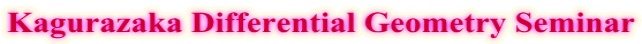To Japanese versionFuture Schedule

Date ： 30 October
Place ： Online by Zoom

Register for participation by Zoom

15:00-16:00
Speaker ： Yuya Takahashi （Nagoya University）
Title : The moduli space of spatial polygons and geometric quantization

Abstract:
The moduli space of spatial polygons is known as a symplectic manifold equipped with both Kahler and real polarizations. In this talk, we will construct morphisms of operads $\mathsf{f}_{Kah}$ and $\mathsf{f}_{re}$ by using the quantum Hilbert spaces $\mathcal{H}_{Kah}$ and $\mathcal{H}_{re}$ associated to the Kahler and real polarizations respectively. Moreover, we will relate the two morphisms $\mathsf{f}_{Kah}$ and $\mathsf{f}_{re}$, and prove the equality
$\dim \mathcal{H}_{Kah} = \dim \mathcal{H}_{re}$ in general setting.
This operadic framework is regarded as a development of the recurrence relation
method by Kamiyama (2000) for proving $\dim\mathcal{H}_{Kah} = \dim \mathcal{H}_{re}$ in a special case.

16:15-17:15
Speaker ： Kurando Baba （Tokyo University of Science）
Title : Calabi-Yau structure and Bargmann type transformation
on the Cayley projective plane
Abstract:

In this talk, I would like to show the existence of Calabi-Yau structure on the punctured cotangent bundle of the Cayley projective plane, and to construct a Bargmann type transformation between a space of holomorphic functions on the bundle and the L2-space on the Cayley projective space. The transformation gives a quantization of the geodesic flow in terms of one parameter group of elliptic Fourier integral operators. This talk is based on a joint work with Kenro Furutani (Osaka City University Advanced Mathematical Institute) arXiv:2101.07505.

Organizers : Naoyuki Koike, Makiko Tanaka,
Akifumi Sako,
Yasufumi Nitta,
Kurando Baba,
Toru Kajigaya, Shunsuke Saito, Tsukasa Takeuchi